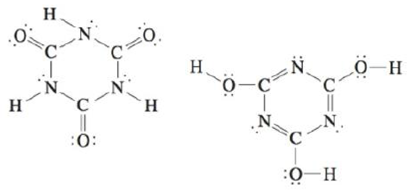# Two structures can be drawn for cyanuric acid: a. Are these two structures the same molecule? Explain. b. Give the hybridization of the carbon and nitrogen atoms in each structure. c. Use bond energies (Table 3-3) to predict which form is more stable; that is, which contains the strongest bonds?### Chemistry: An Atoms First Approach

2nd Edition
Steven S. Zumdahl + 1 other
Publisher: Cengage Learning
ISBN: 9781305079243

#### Solutions

Chapter
Section### Chemistry: An Atoms First Approach

2nd Edition
Steven S. Zumdahl + 1 other
Publisher: Cengage Learning
ISBN: 9781305079243
Chapter 4, Problem 85AE
Textbook Problem
27 views

## Two structures can be drawn for cyanuric acid:a. Are these two structures the same molecule? Explain.b. Give the hybridization of the carbon and nitrogen atoms in each structure.c. Use bond energies (Table 3-3) to predict which form is more stable; that is, which contains the strongest bonds?

(a)

Interpretation Introduction

Interpretation: The answers are to be stated for the given two structures.

Concept introduction: The hybridization of an atom can be obtained by finding its steric number. The sum of the numbers of atoms bonded to the required atom and the number of lone pairs the atom has is known as the steric number.

If the steric number is 4 , the atom is sp3 hybridized.

If the steric number is 3 , the atom is sp2 hybridized.

If the steric number is 2 , the atom is sp hybridized.

Bond strength can be determined by bond energy.

To determine: The given structures are same or not.

### Explanation of Solution

The given structures are

The given structures are not same because

• In structure (I), functional group is C=O

(b)

Interpretation Introduction

Interpretation: The answers are to be stated for the given two structures.

Concept introduction: The hybridization of an atom can be obtained by finding its steric number. The sum of the numbers of atoms bonded to the required atom and the number of lone pairs the atom has is known as the steric number.

If the steric number is 4 , the atom is sp3 hybridized.

If the steric number is 3 , the atom is sp2 hybridized.

If the steric number is 2 , the atom is sp hybridized.

Bond strength can be determined by bond energy.

To determine: The hybridizations of carbon and nitrogen atoms.

(c)

Interpretation Introduction

Interpretation: The answers are to be stated for the given two structures.

Concept introduction: The hybridization of an atom can be obtained by finding its steric number. The sum of the numbers of atoms bonded to the required atom and the number of lone pairs the atom has is known as the steric number.

If the steric number is 4 , the atom is sp3 hybridized.

If the steric number is 3 , the atom is sp2 hybridized.

If the steric number is 2 , the atom is sp hybridized.

Bond strength can be determined by bond energy.

To determine: The comparison of stability of given structures.

### Still sussing out bartleby?

Check out a sample textbook solution.

See a sample solution

#### The Solution to Your Study Problems

Bartleby provides explanations to thousands of textbook problems written by our experts, many with advanced degrees!

Get Started

Find more solutions based on key concepts
Which of these foods should form the bulk of the pregame meal? a. breads, potatoes, pasta, and fruit juices. b....

Nutrition: Concepts and Controversies - Standalone book (MindTap Course List)

How is temperature related to the density of air?

Fundamentals of Physical Geography

Bacteria, Archaea, and Eukarya are three ________.

Biology: The Unity and Diversity of Life (MindTap Course List)

What are the moral implications of using alcohol during pregnancy?

Understanding Nutrition (MindTap Course List)

What is the fuel density in units of kg/L?

Chemistry & Chemical Reactivity

6.45 Define the term shielding.

Chemistry for Engineering Students

Define the following terms: a. chromosome b. chromatin

Human Heredity: Principles and Issues (MindTap Course List)

Figure 15.4 shows two curves representing particles undergoing simple harmonic motion. The correct description ...

Physics for Scientists and Engineers, Technology Update (No access codes included)

Why are no chondrules seen in achondritic meteorites?

Foundations of Astronomy (MindTap Course List)

In what ways did the work of Alfred Thayer Mahan influence the history of the 20th century?

Oceanography: An Invitation To Marine Science, Loose-leaf Versin